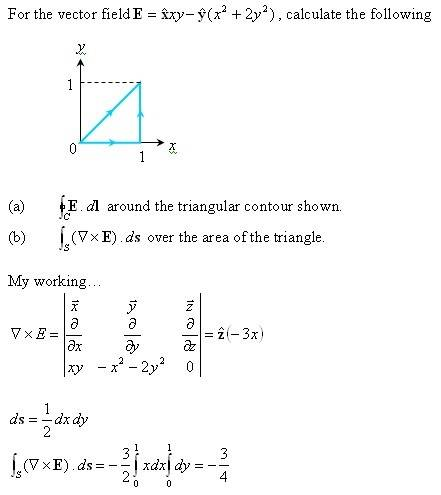# Stoke's Theorem.

Hi guys... I'm stuck in this question. Its not a homework nor coursework, just practice. The answer for both is the same, that is -1 ...I'm able to get (a) but (b) .... still wondering what went wrong. Please enlighten me. Thanks in advance.Last edited:

quasar987
Homework Helper
Gold Member
That ds=dxdy/2 part.. it's not how it works. what if I wanted to integrate over a circle? would you take ds=pi (dx²+dy²)?

chickens said:
Hi guys... I'm stuck in this question. Its not a homework nor coursework, just practice. The answer for both is the same, that is -1 ...I'm able to get (a) but (b) .... still wondering what went wrong. Please enlighten me. Thanks in advance.
View attachment 8120

Also, how can you say this:
ds = 1/2dxdy

You are saying that ds is a vector. Yet, 1/2dxdy is not.

sorry... that ds suppose to have z_hat with it

quasar987 said:
That ds=dxdy/2 part.. it's not how it works. what if I wanted to integrate over a circle? would you take ds=pi (dx²+dy²)?

i'm not sure about the ds part as usually there is this table i would refer to, and it says ds = dxdy z_hat but ... i've also tried doing using it, i wont get the same answer.

If need to integrate over a circle, transform the vector to cylindrical type and use cylindrical variables r phi z to get it?

In tutorial classes, they gave a semicircle and that was quite easy to get it.... but this geometrical shape triangle.... is my first time trying it, and i got so say... its cracking my head (i know its simple stuff...but i really dont get where went wrong)

HallsofIvy
Homework Helper
First, at least one of the arrows in your picture is going the wrong way. I suspect that your contour is supposed to go FROM (1, 1) T0 (0, 0), not the otherway round as you have it.

$d\vec{S}$ is NOT "$\frac{1}{2} dxdy \vec{z}$". The fact that you are integrating over a triangle has nothing to do with the differential of area (everything to do with limits of integration!). Since this is in the xy-plane, $d\vec{S}= dxdy \vec{z}$. The integral is NOT for $0\le x\le 1$, $0\le y\le 1$; that would be over the unit square. You want to integrate over the triangle with boundaries y= 0, x= 1, y= x.

For part (b) break the triangle into the three sides. Write parametric equations for each.

HallsofIvy said:
First, at least one of the arrows in your picture is going the wrong way. I suspect that your contour is supposed to go FROM (1, 1) T0 (0, 0), not the otherway round as you have it.

$$d\vec{S}$$ is NOT "$$\frac{1}{2} dxdy \vec{z}$$". The fact that you are integrating over a triangle has nothing to do with the differential of area (everything to do with limits of integration!). Since this is in the xy-plane, $$d\vec{S}= dxdy \vec{z}$$. The integral is NOT for $$0\le x\le 1$$, $$0\le y\le 1$$; that would be over the unit square. You want to integrate over the triangle with boundaries y= 0, x= 1, y= x.

For part (b) break the triangle into the three sides. Write parametric equations for each.

yup...sorry... the contour suppose to go from (1,1) to (0,0) thanks for the mistake pointed out :)

anyway...mind reedit the latex thing... cant seem to get them =_=